Miscellaneous

Chapter 11 Class 12 Three Dimensional Geometry
Serial order wise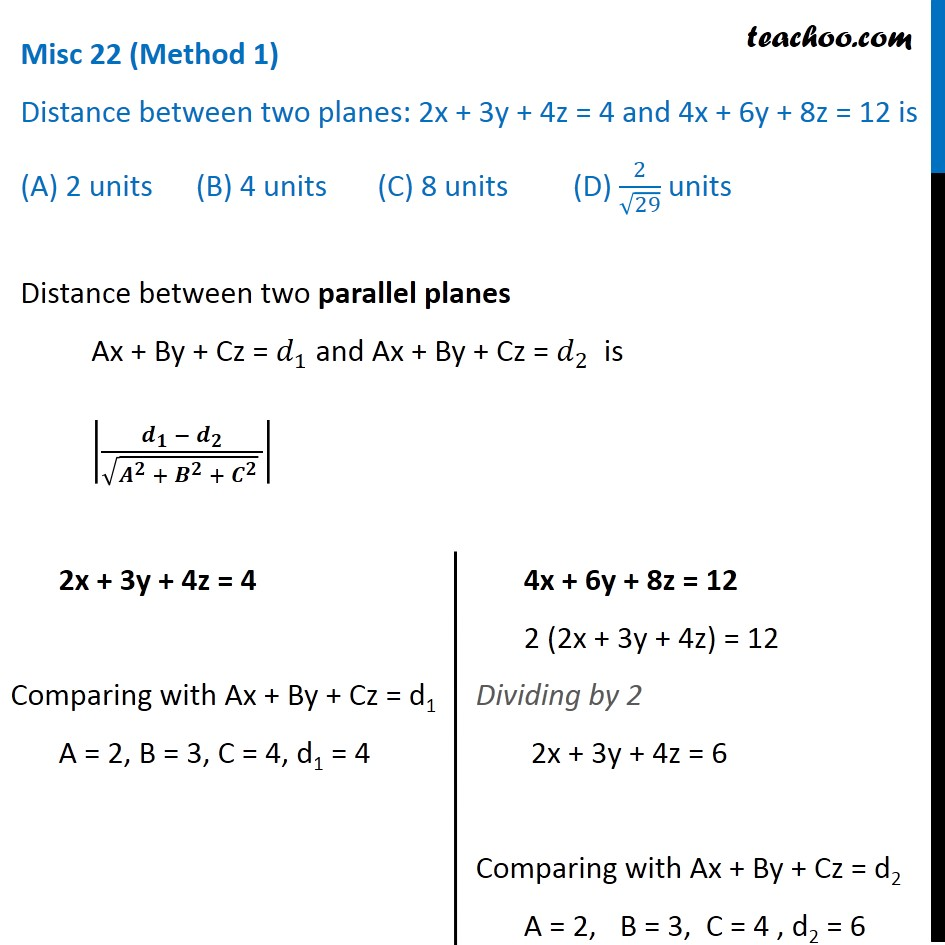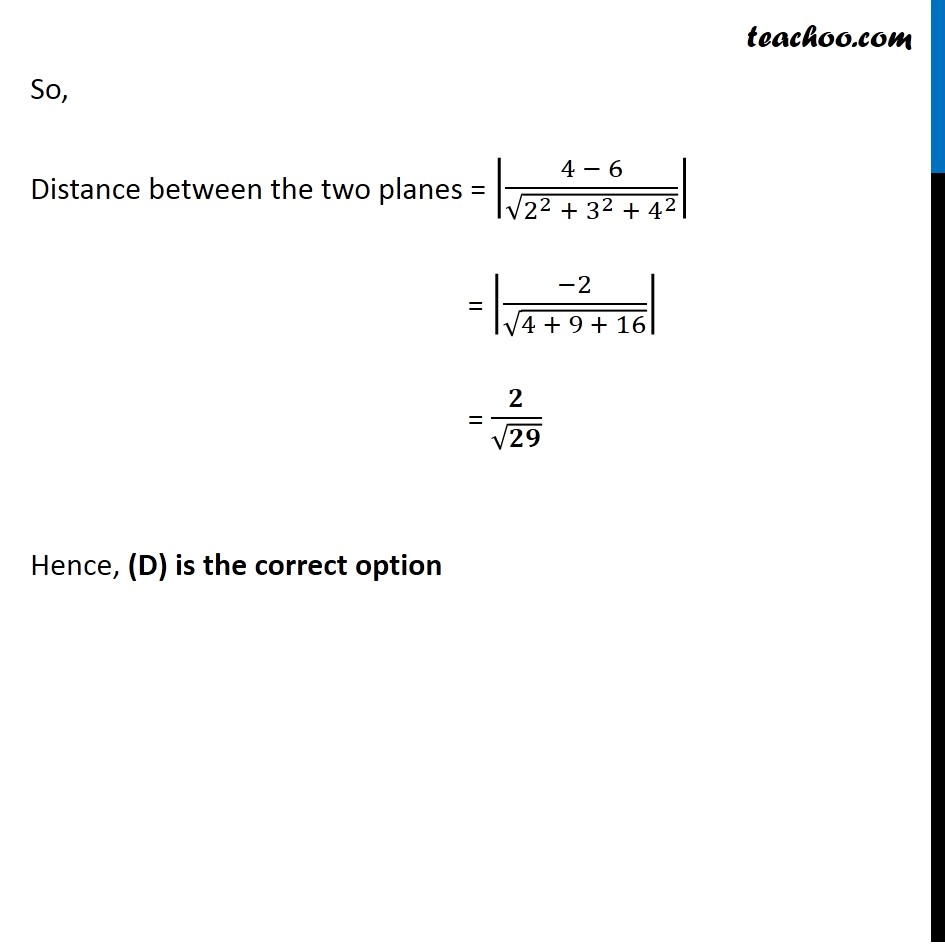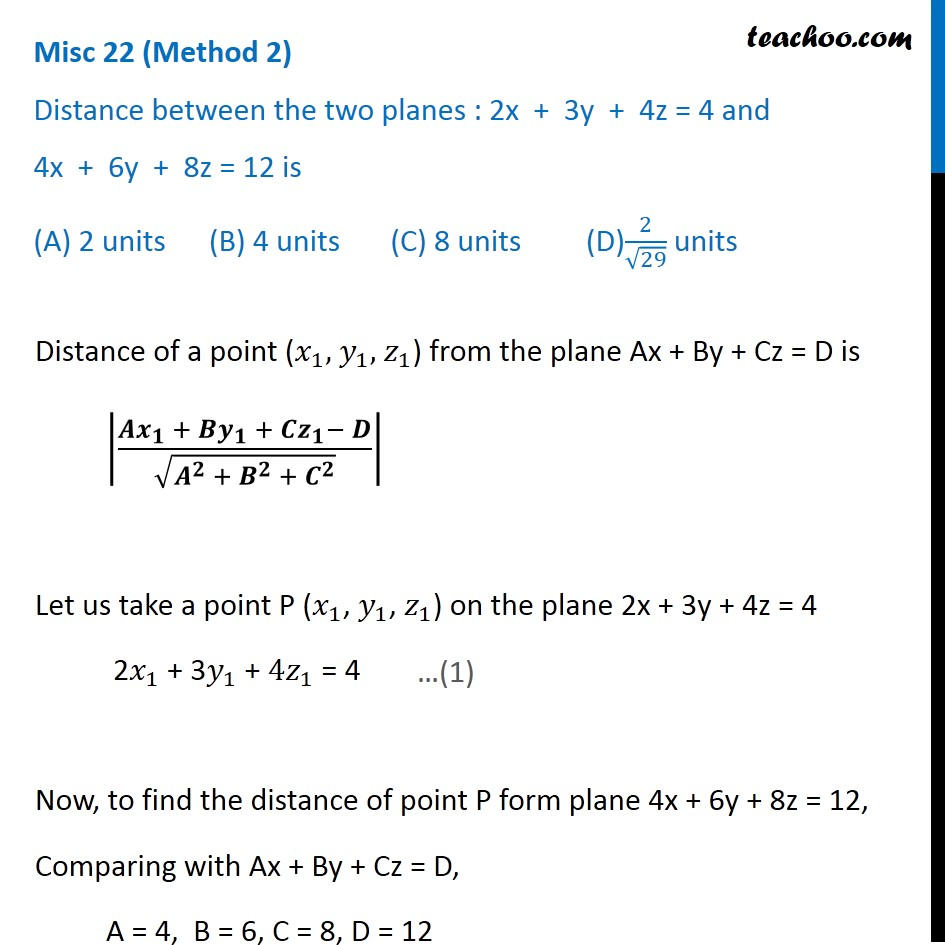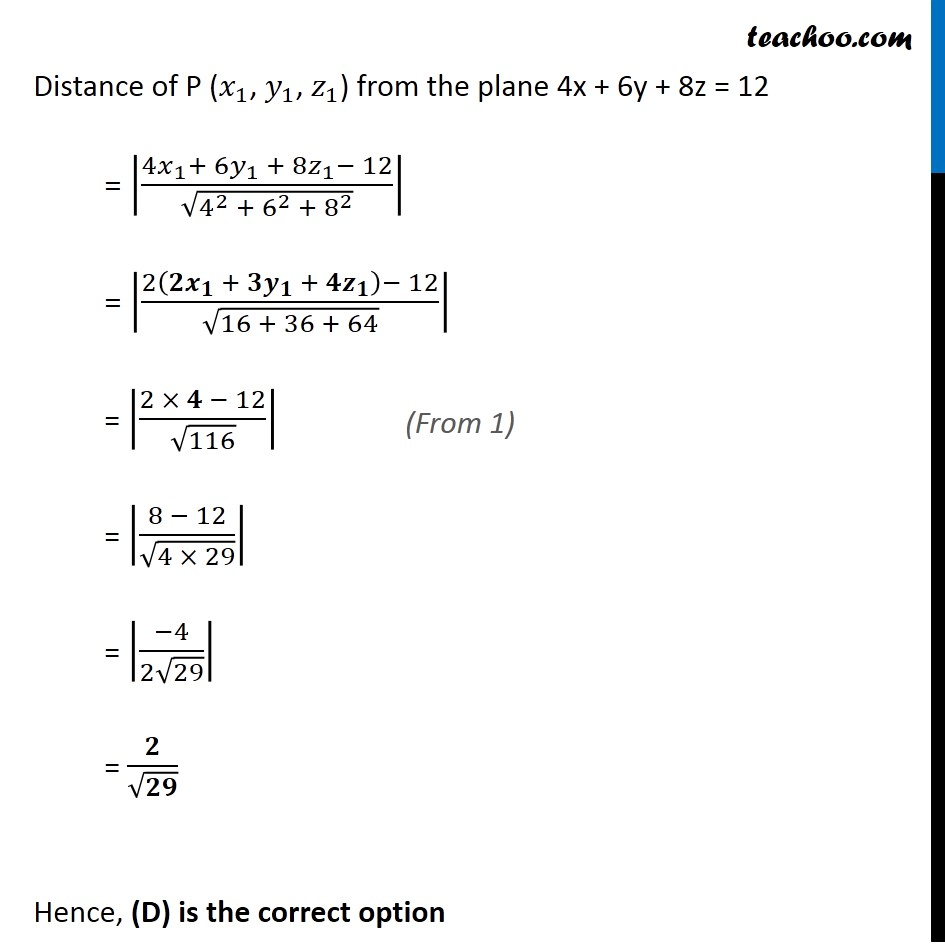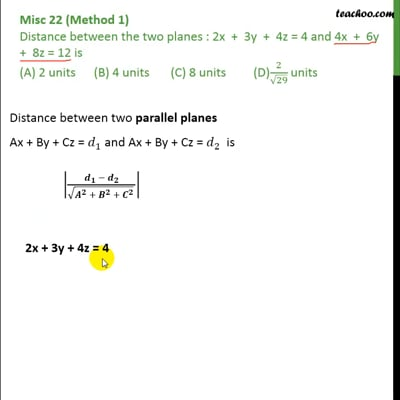This video is only available for Teachoo black users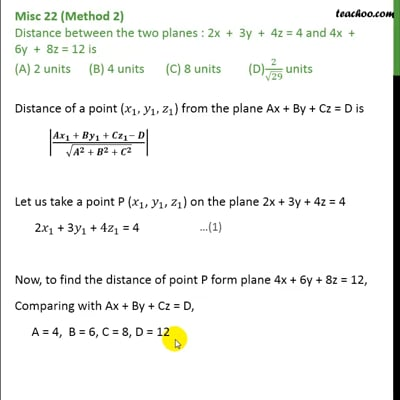This video is only available for Teachoo black users

Solve all your doubts with Teachoo Black (new monthly pack available now!)

### Transcript

Misc 22 (Method 1) Distance between two planes: 2x + 3y + 4z = 4 and 4x + 6y + 8z = 12 is (A) 2 units (B) 4 units (C) 8 units (D) 2/√29 units Distance between two parallel planes Ax + By + Cz = 𝑑_1 and Ax + By + Cz = 𝑑_2 is |(𝒅_𝟏 − 𝒅_𝟐)/(√(𝑨^𝟐 + 𝑩^𝟐 + 𝑪^𝟐 ) )| 2x + 3y + 4z = 4 Comparing with Ax + By + Cz = d1 A = 2, B = 3, C = 4, d1 = 4 4x + 6y + 8z = 12 2 (2x + 3y + 4z) = 12 Dividing by 2 2x + 3y + 4z = 6 Comparing with Ax + By + Cz = d2 A = 2, B = 3, C = 4 , d2 = 6 So, Distance between the two planes = |(4 − 6)/√(2^2 + 3^2 + 4^2 )| = |(−2)/√(4 + 9 + 16)| = 𝟐/√𝟐𝟗 Hence, (D) is the correct option Misc 22 (Method 2) Distance between the two planes : 2x + 3y + 4z = 4 and 4x + 6y + 8z = 12 is (A) 2 units (B) 4 units (C) 8 units (D)2/√29 units Distance of a point (𝑥_1, 𝑦_1, 𝑧_1) from the plane Ax + By + Cz = D is |(𝑨𝒙_𝟏 + 𝑩𝒚_𝟏 + 𝑪𝒛_𝟏− 𝑫)/√(𝑨^𝟐 + 𝑩^𝟐 + 𝑪^𝟐 )| Let us take a point P (𝑥_1, 𝑦_1, 𝑧_1) on the plane 2x + 3y + 4z = 4 2𝑥_1 + 3𝑦_1 + 4𝑧_1 = 4 Now, to find the distance of point P form plane 4x + 6y + 8z = 12, Comparing with Ax + By + Cz = D, A = 4, B = 6, C = 8, D = 12 Distance of P (𝑥_1, 𝑦_1, 𝑧_1) from the plane 4x + 6y + 8z = 12 = |(4𝑥_1+ 〖6𝑦〗_1 + 8𝑧_1− 12)/√(4^2 + 6^2 + 8^2 )| = |(2(𝟐𝒙_𝟏 + 〖𝟑𝒚〗_𝟏 + 𝟒𝒛_𝟏 )− 12)/√(16 + 36 + 64)| = |(2 × 𝟒 − 12)/√116| = |(8 − 12)/√(4 × 29)| = |(−4)/(2√29)| = 𝟐/√𝟐𝟗 Hence, (D) is the correct option